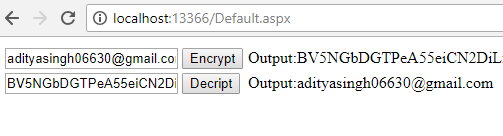Introduction:

In this article we are going to explain how to Encrypt and Decrypt website URL or Email Address in Asp.net with example.

Description:

Encrypt and decrypt are very important data in asp.net For security purpose, we are storing some valuable things in Encrypt format.

Let’s see an example:

```using System;
using System.Collections.Generic;
using System.IO;
using System.Linq;
using System.Security.Cryptography;
using System.Text;
using System.Web;
using System.Web.UI;
using System.Web.UI.WebControls;

namespace TutorialsAsp.Net
{
public partial class Default : System.Web.UI.Page
{
protected void Page_Load(object sender, EventArgs e)
{

}

public string Encrypt(string inputText)
{
string encryptionkey = "ACDS193BX628TD57";
byte[] keybytes = Encoding.ASCII.GetBytes(encryptionkey.Length.ToString());
RijndaelManaged rijndaelCipher = new RijndaelManaged();
byte[] plainText = Encoding.Unicode.GetBytes(inputText);
using (ICryptoTransform encryptrans = rijndaelCipher.CreateEncryptor(pwdbytes.GetBytes(32), pwdbytes.GetBytes(16)))
{
using (MemoryStream mstrm = new MemoryStream())
{
using (CryptoStream cryptstm = new CryptoStream(mstrm, encryptrans, CryptoStreamMode.Write))
{
cryptstm.Write(plainText, 0, plainText.Length);
cryptstm.Close();
return Convert.ToBase64String(mstrm.ToArray());
}
}
}
}
public string Decrypt(string encryptText)
{
string encryptionkey = "ACDS193BX628TD57";
byte[] keybytes = Encoding.ASCII.GetBytes(encryptionkey.Length.ToString());
RijndaelManaged rijndaelCipher = new RijndaelManaged();
byte[] encryptedData = Convert.FromBase64String(encryptText.Replace(" ", "+"));
using (ICryptoTransform decryptrans = rijndaelCipher.CreateDecryptor(pwdbytes.GetBytes(32), pwdbytes.GetBytes(16)))
{
using (MemoryStream mstrm = new MemoryStream(encryptedData))
{
using (CryptoStream cryptstm = new CryptoStream(mstrm, decryptrans, CryptoStreamMode.Read))
{
byte[] plainText = new byte[encryptedData.Length];
int decryptedCount = cryptstm.Read(plainText, 0, plainText.Length);
return Encoding.Unicode.GetString(plainText, 0, decryptedCount);
}
}
}
}

protected void btnEncrypt_Click(object sender, EventArgs e)
{
lblEncrypt.Text = Encrypt(txtEncrypt.Text);
}

protected void btnDecrypt_Click(object sender, EventArgs e)
{
lblDecrypt.Text = Decrypt(txtDecrypt.Text);
}

}
}
```

Output:I hope this article will help to you after reading.

Modified On Sep-07-2019 12:18:13 AM